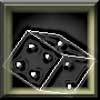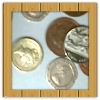#### You may also like### Gambling at Monte Carlo

A man went to Monte Carlo to try and make his fortune. Is his strategy a winning one?### Marbles and Bags

Two bags contain different numbers of red and blue marbles. A marble is removed from one of the bags. The marble is blue. What is the probability that it was removed from bag A?### Win or Lose?

A gambler bets half the money in his pocket on the toss of a coin, winning an equal amount for a head and losing his money if the result is a tail. After 2n plays he has won exactly n times. Has he more money than he started with?

# Coin Tossing Games

##### Age 14 to 16Challenge Level

The games involve tossing a fair coin until my chosen sequence or your chosen sequence occurs.

If I pick HH and you pick TH the only way that I can win is if the first two throws are HH. This occurs with probability 1/4 so P(you win) = 3/4.

What if I pick HH and you pick HT ?

If the first two throws are HH then I win, and if they are HT then you win. If we get TH, then the third throw is equally likely to be H (I win) or T (you win), so if the first pair is TH, then the probability that you win is 1/2. If the first two throws are TT let p be the probability that you win. The third throw is equally likely to be H (we have seen that the probability that you win starting from TH is 1/2 ) or T (and the probability that you win from TT is p). Thus

p = (1/2 x 1/2) + (p x 1/2) so that p = 1/2.

This leads to P(you win)}= (0 + 1 + 1/2 + 1/2 )/4 = 1/2.

What if I pick HH and you pick TT ?

If the first pair is HH then I win, and if the first pair is TT then you win. Let p and r be the probability that you win if the first pair is HT and TH respectively. By considering the outcome of the third throw, we get

p = 1/2 + (r x 1/2)

r = p x 1/2

Solving these equations, we find p=2/3 and r=1/3.
This means that P(you win) = (0 + 1 + 2/3 + 1/3)/4 = 1/2.

What is the best choice you can make?
The best choice you can make is TH.

What should you choose if I choose TT ?
By symmetry between H and T, if I choose TT then you should choose HT, and then P(you win = 3/4.

What happens if I choose HT ?

 1 We have already considered HH versus HT and found that they are equally likely to win. So if I choose HT and you choose HH , then the probability that you win is 1/2. 2 Suppose you choose TH. If the first pair is HH or HT then I win, and if it is TH or TT then you win, and so P(you win)=1/2. 3 Suppose that you choose TT. We have already considered TT versus HT , and we know that the probability that HT wins is 3/4. Hence the probability that you win with TT against HT is 1/4.

Assuming that you always make a choice that maximises your chance of winning, what should I choose to maximise the probability that I win?

We still have to consider what happens if I choose TH.
By symmetry with the case where I choose HT , we see that if you choose HH then you win with probability 1/4, if you choose HT then you win with probability 1/2, and if you choose TT then you win with probability 1/2.

Using all the above results, I should choose HT or TH, because either of these choices makes my probability of winning equal to 1/2, whereas for HH and TT my winning probability is only 1/4 (assuming that you always make a choice that maximises your chance of winning).

Now suppose that we look at triples instead of pairs.
What is the probability that you win if I choose HHH and you choose THH ?

I can only win if the first three throws are HHH.
This occurs with probability 1/8 , so the probability that you win is 7/8.

I have eight possible choices and, for each one, you can find a triple that gives you a better than even chance of winning (ie a triple that makes your probability of winning more that 1/2).

We have seen just above that it is possible if I choose HHH. We now consider all the other possibilities in turn.

 1 If I choose TTT then by symmetry you should choose HTT so that your probability of winning is 7/8. 2 I choose HTH . Suppose you try HHT. You win if the starting triple is HHH , HHT or THH. I win if the starting triple is HTH. Let p , q , r and s be the probability that you win if the starting triple is HTT , THT , TTH and TTT respectively.

By considering what happens at the fourth throw, we find

p = (r x 1/2) + (s x 1/2)
q = (p x 1/2)
r = (qx1/2) + 1/2
s = (r x 1/2) + (s x 1/2).

We find r = s = p = 2/3 and q = 1/3.
This means that
P(you win) = (1 + 1 + 0 + 2/3 + 1 + 1/3 + 2/3 + 2/3)/8 = 2/3.

 3 By symmetry, if I choose THT and you choose TTH,then P(you win) = 2/3. 4 I choose HHT. Suppose you try choosing THH. I win if the starting triple is HHH or HHT , otherwise you win, so that {P(you win) = 3/4. 5 By symmetry, if I choose TTH and you choose HTT , then P(you win) = 3/4. 6 I choose HTT. Suppose you choose HHT . Then you win if the starting triple is HHH , HHT or THH , but I win if it is HTT . Carrying out calculations similar to those above, we find that if the starting triple is HTH , TTH or TTT then your winning probability is 2/3, but if it is THT your winning probability is 1/3. Thus P(you win) = 2/3. 7 By symmetry, if I choose THH and you choose TTH, then P(you win) = 2/3.

For each of my eight possible choices, there is a triple that gives you a better than even chance of winning.